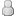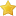• 11 Votes - 4.64 Average
• 1
• 2
• 3
• 4
• 5
10-17-2016, 06:16 AMPost: #280
 LunaMooPosting FreakPosts: 1,706 Joined: Sep 2013 Reputation: 157
"xBRZ" is not a shader, but a cpu scaler which ppsspp uses for texture scaling, you can select it's scale in options, but it doesn't really have anything to do with shaders.

If you however ment to write "2xBR" then sure, it's pretty much a variant of 5xBR, added it as an option to my custom shader just now, if you want a separate 2xBR, just grab 5xBR from PPSSPP find and replace this line on the end:
Code:
`    vec3 res = nc.x ? px.x ? F : H : nc.y ? px.y ? B : F : nc.z ? px.z ? D : B : nc.w ? px.w ? H : D : E;`
with all of this code:
Code:
```    vec3 E0 = E.xyz;     vec3 E1 = E.xyz;     vec3 E2 = E.xyz;     vec3 E3 = E.xyz;     vec3 P;     P = px.x ? F : H;     P = px.y ? B : F;     P = px.z ? D : B;     P = px.w ? H : D;     if (edr.x) {         if (edr_left.x && edr_up.x) {             E3  = mix(E3 , P,  0.833333);             E2  = mix(E2 , P,  0.25);             E1  = mix(E1 , P,  0.25);         } else if (edr_left.x) {             E3  = mix(E3 , P,  0.75);             E2  = mix(E2 , P,  0.25);         } else if (edr_up.x) {             E3  = mix(E3 , P,  0.75);             E1  = mix(E1 , P,  0.25);         } else {             E3  = mix(E3 , P,  0.5);         }     }     if (edr.y) {         if (edr_left.y && edr_up.y) {             E1  = mix(E1 , P,  0.833333);             E3  = mix(E3 , P,  0.25);             E0  = mix(E0 , P,  0.25);         } else if (edr_left.y) {             E1  = mix(E1 , P,  0.75);             E3  = mix(E3 , P,  0.25);         } else if (edr_up.y) {             E1  = mix(E1 , P,  0.75);             E0  = mix(E0 , P,  0.25);         } else {             E1  = mix(E1 , P,  0.5);         }     }     if (edr.z) {         if (edr_left.z && edr_up.z) {             E0  = mix(E0 , P,  0.833333);             E1  = mix(E1 , P,  0.25);             E2  = mix(E2 , P,  0.25);         } else if (edr_left.z) {             E0  = mix(E0 , P,  0.75);             E1  = mix(E1 , P,  0.25);         } else if (edr_up.z) {             E0  = mix(E0 , P,  0.75);             E2  = mix(E2 , P,  0.25);         } else {             E0  = mix(E0 , P,  0.5);         }     }     if (edr.w) {         if (edr_left.w && edr_up.w) {             E2  = mix(E2 , P,  0.833333);             E0  = mix(E0 , P,  0.25);             E3  = mix(E3 , P,  0.25);         } else if (edr_left.w) {             E2  = mix(E2 , P,  0.75);             E0  = mix(E0 , P,  0.25);         } else if (edr_up.w) {             E2  = mix(E2 , P,  0.75);             E3  = mix(E3 , P,  0.25);         } else {             E2  = mix(E2 , P,  0.5);         }     }     vec3 res = (fp.x < 0.50) ? (fp.y < 0.50 ? E0 : E2) : (fp.y < 0.50 ? E1: E3);```
and it will turn into 2xBR, should look exactly same on lower display resolutions.

No idea what you mean by overlay shader, if by PNG you ment texture in-game - PPSSPP only supports simple "postprocessing" shaders, the only "texture" those sees is the output.